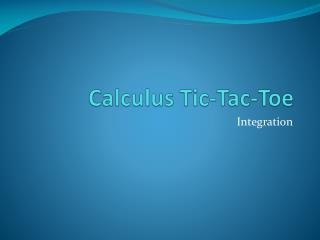# Calculus Tic- Tac -Toe - PowerPoint PPT PresentationDownload PresentationCalculus Tic- Tac -Toe

Calculus Tic- Tac -ToeDownload Presentation## Calculus Tic- Tac -Toe

- - - - - - - - - - - - - - - - - - - - - - - - - - - E N D - - - - - - - - - - - - - - - - - - - - - - - - - - -
##### Presentation Transcript

1. Calculus Tic-Tac-Toe Integration

2. First some review… • To integrate, you must have a sum/difference or constant multiple combination of a variable to a number power (xn). • You may need to substitute one function for another in order to manipulate the integral to be in this form! • Integration finds net area between curves by accumulating heights.

3. Now you’re ready to play! • Get into the following groups of students – move your desks into these groups!!

4. Now you’re ready to play! • Designate one person in your group to “keep score”. This person should draw a blank Tic-Tac-Toe board on a piece of paper. Keep track of which spaces you have filled in on this board by marking an X for the ones you get correct and an O for the ones do you not. • Remember: Your goal is to make three X’s across, down, or diagonally (just like in normal Tic-Tac-Toe). First group to accomplish this, wins 10 bonus points!

5. Choose one square of each type to make Tic-Tac-Toe…

6. Comprehension Integrate: (Do not click the option below until EVERYONE has attempted the problem!) • Answer

7. Comprehension Answer • Discuss as a class why the answer is negative. • Hint: Think about the location of the graph of cosx between π/2 and π. • Back to Game Board

8. Comprehension Integrate: (Do not click the option below until EVERYONE has attempted the problem!) • Answer

9. Comprehension Answer • Back to Game Board

10. Comprehension Integrate (Do not click an option below until EVERYONE has attempted the problem!) • Answer

11. Comprehension Answer • Back to Game Board

12. Creative Thinking Integrate (Do not click the option below until EVERYONE has attempted the problem!) • Answer

13. Creative Thinking Answer • Back to Game Board

14. Creative Thinking Integrate (Do not click the option below until EVERYONE has attempted the problem!) • Answer

15. Creative Thinking Answer • Back to Game Board

16. Creative Thinking Integrate (Do not click the option below until EVERYONE has attempted the problem!) • Answer

17. Creative Thinking Answer • Back to Game Board Recall…

18. Application • The graph of f(x) is shown: • Calculate (Do not click the option below until EVERYONE has attempted the problem!) • Answer

19. Application Answer • Recall…the area above the x-axis is positive and the area below the x-axis is negative in integration. • Using geometric shapes, divide the positive region into a triangle from [-1,0], a rectangle from [0,1], and a triangle from [1,2]. • Add these up! 1+2+1 = 4 • Subtract the triangular region from [2,3] and the rectangular region from [3,4]. • 4-.5-1 = 2.5 • Back to Game Board

20. Application • The function f is continuous for all 1 < x < 7. • Approximate the value of using MRAM with 3 equal subdivisions. (Do not click the option below until EVERYONE has attempted the problem!) • Answer

21. Application Answer • Since we need 3 equal subdivisions, use: A1= the area from [1,3], ∆x = 2 A2= the area from [3,5], ∆x = 2 and A3= the area from [5,7], ∆x = 2. A1= (2)(f(2)) = 2(2) = 4 A2= (2)( f(4))= 2(2) = 4 A3= (2)( f(6)) = 2(-1) = -2 A1+ A2+ A3 = 4+4+-2 = 6. • Back to Game Board

22. Application • The temperature T (in ˚C) recorded during a day followed the curve where t is time and noon is t = 0. (In other words, for this day, -12 ≤ t ≤ 12.) • What was the average temperature during the day? (Do not click the option below until EVERYONE has attempted the problem!) • Answer

23. Application Answer • For average temperature, “add up all the temperatures and divide by the number of temperatures accumulated.” • Back to Game Board